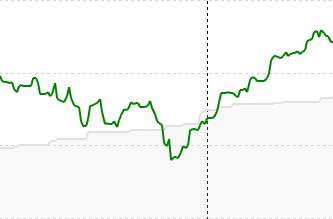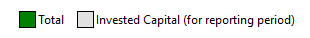# Graphs of investment and performance

Hi,
how can I get a graph that shows simultaneosly the investments and the time-weighted performance? See the example below.

That would be much appreciated.
thanks

You can’t, because one is a currency amount and one is a percentage. You can, however, put two separate graphs next to each other.

Maybe I’ve used incorrect words, but I m referring to plot two values both in currency amount.

One is the actual amount invested (red line), the other is the amount invested plus the TWR (time weighted rate of return) that should be a currency amount too (blue line).

For instance, in the graph I’ve plotted I can see that TWR is negative and how much.

If your able to explain, how to convert the TTWROR percentage into the corresponding amount…

It is not. As I said, and as the term rate of return implies, it is a percentage.

No guys, don’t get me wrong.

As I said my words may be incorrect, but I just would like to know if it is possible to plot a graph whit the money invested and their actual value. I don’t know which are the correct technical names.

I’ve posted the example plot because an image it s worth a thousand words.

Thank you

You simply mean something like that?You can find that at
Reports > Statement of Assets > Chart
where you can create a chart and add the two data series shown in the screenshot.

1 Like

Not exactly, I would like to plot both the ‘deposit’ graph and the actual value of the assets in which that deposit has been invested.

In ‘chart’ I cannot find a way to plot the deposit curve.

1 Like

Invested capital is something very close to what I’m looking for.

I just wanted to show this for each security accounts. Is this feasible?

Thank you

Invested capital is what is displayed in my screenshot too. But it is always the invested capital for the total portfolio. You can not display invested capital only for a specific security account.

Such a feature (selecting a security account first instead of the total portfolio and then choose the data like invested capital) was discussed in german here:

At the moment there is no such feature.

2 Likes